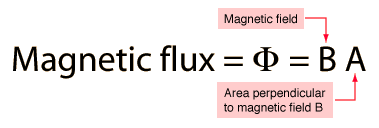﻿ What is Flux??? Types of Flux?Back

## What is Flux??? Types of Flux?# What is Flux? Types of Flux?

Meaning of Flux:

Flux means an imaginary line through which a physical quantity can travel. The word “Flux” is originated from Latin Word ‘Fluxus’ that means flow. Isaac Newton first used this term as fluxion into differential calculus. In mathematics and physics flux is used as the basic and global word.Types of Flux:

Flux can be used in various concepts, such as,#### 1) Magnetic Flux

It means the number magnetic field lines passing through a closed surface. Its SI unit is – Weber and in CGS is – Maxwell. It is denoted as Φm.

2) Electric Flux

It means the number of electric field lines passing through a closed surface. Its SI unit is – volt-meter. It is denoted as ΦE.

3) Luminous Flux

It means the line of transmitted visually sensible radiated energy per second. Its SI unit is Lumen (lm).

It means the line of transmitted radiated energy per second from any energy source. Its SI unit is Watt. It is denoted as ΦV.

4) Heat Flux

It means the heat energy transfer through an elementary surface per second. Its SI unit is W/m2. It is denoted as Φq.

5) Mass Flux

It means the rate of mass flow per unit area. Its SI unit is kg/s-m2. It is denoted as J or q.

6) Momentum Flux

It means flow of momentum across unit area per second. Its SI unit is N/m2.

7) Acoustic Flux

It means flow of sound energy through unit area per second. Its SI unit is Watt.